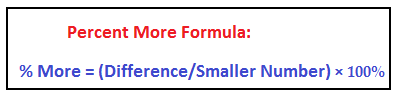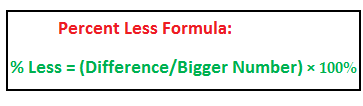## Percentages

What is the chance heads will appear on tossing a dice?

Answer: 50%.

It is read as “Fifty per cent” symbolically written as “50%”.The percent symbol “%” is the fraction: 1/100

i.e., 50% is 50 × (1/100) = 50/100 = ½

Per cent means per hundred.

Cent is centum i.e., 100.

The symbol % denotes percent.

Examples:

• Eighty percent is written as 80%,
• Hundred percent is 100%
• Hundredth percent is (1/100)%

1. To Convert Percentage into Fractions:

Method:

Write 100 in denominator for the percentage symbol: %

Examples:

Convert 50%, 80% and 100% into fractions.

50% = 50/100 = ½,

80% = 80/100 = 8/10 = 4/5,

100% = 100/100 = 1

2. To Convert Fractions into Percents:

Method:

Multiply the given fraction with 100 and write % (the percentage symbol) beside the simplified number.

Examples:

1. ½ is ½ × 100% = 50%

2. 1/3 is 1/3 × 100% = 33.33%

3. ¼ is ¼ × 100% = 25%

3. The Five Important Types of Questions in Percents:

1.Percent Increase:

Example:

The price of a book rises from \$10 to \$12. What is the percent increase in the price?

Solution:

Price rises by 2.

A \$2 increase on \$10 is how much increase on hundred, i.e how much increase per cent?

2 on 10 is how much on 100 i.e., per hundred:

(2/10) × 100% = 20%In the above example:

Initial value is 10, Final value is 12 and so increase is 12 – 10 = 2.

Therefore, % increase = (2/10) × 100 % = 20%

2. Percent Decrease:

Example:

The price of book decreased from \$12 to \$10. What is the percent decrease in the price?

Solution:

The price decreased from \$12 to \$10 i.e., by \$2.

\$2 decrease on 12 is how much decrease on 100 i.e. per cent?

2 on 12 is how much per 100:

(2/12) × 100% = (1/6) × 100% = 16.66%3. How much percent is one thing more than another thing?

Example:

In a test, Jim scored 40, and Kim scored 30. By what percent Jim scored more than Kim?

Solution:

Jim’s score 40 is 10 more than Kim’s score 30.

40 is 10 more than 30.

10 more than 30 is how much more than hundred or

10 more on 30 is how much more per cent?

(10/30) × 100% = 33.33%

Note:

A question on % More signifies comparison of two quantities. Here 40 and 30. And in comparison normally, one is Bigger and the other, Smaller.

So, for the solution on % more, write the smaller number in the denominator.4. How much percent is one thing less than another thing?

Example:

In a test, Jim scored 40 and Kim scored 30. How much percent is Kim’s score less than Jim’s?

Solution:

Kim’s score 30 is 10 less than Jim’s score 40

30 is 10 less than 40, then how much is 30 less than hundred i.e., per cent?

10 less than 40 is how much less per cent:

(10/40) × 100% = 25%Note:

A question on % Less signifies comparison of two quantities. Here 40 and 30. And in comparison normally, one is Bigger and the other, smaller.

So, in the solution for % less, write the bigger number in the denominator.

Very Important Clarification:

A widely prevalent mistake is the thought that:

If Jim scores 25% more than Kim, then Kim scores 25% less than Kim too.

But this is obviously mistaken.

Students will do well to carefully note from the above two formulas for % More and % Less that the two formulas do not contain the same number in the denominator.

For % less, the bigger number is written in the denominator and for % more, the smaller.

Take Kim’s score as 100. Now, Jim’s score is 125, i.e. 25% more than Kim’s.

Then % Less = (25/125) × 100 % = 20%

Therefore, if Jim’s score is 25% more than Kim’s, then Kim’s is 20% less than Jim’s score, and not 25%.5. Net percent change in successive percent changes:

Example:

A shirt costs \$40. The price was increased by 20%.

Increase in price is 25% of 40, i.e. (25/100) × 40 = 10.

So, new price is 40 + 10 = 50

Again, the price is increased by another 25%.

Second increase in price is 25% of 50, i.e. (25/100) × 50 = 12.5

Therefore, the final price after the two successive percent changes is

50 + 12.5 = 62.5

Total percent increase in price is:

Initial price = 40, Final price = 62.5, Increase in price = 22.5

Applying the % increase formula,

% increase = (12.5/40) × 100% = 56.25%

Short-cut to find the Net Percent change during successive percent changes:Solve the above example with the short-cut:

Net percent change is

{25 + 25 + (25 × 25)/100}% = (50 + 6.25) % = 56.25%

Note:

1. When there are three successive percent changes, the net percent change from the first two is x and third percent change is y:

Example:

if a price is increased 25% thrice successively, then the net percent change from the first two 25% changes is 56.25%, taken as x and third 25% will then be taken as y.

2. in the above formula, write a ― sign wherever there is a decrease.

+ Sign for increase and ― sign for decrease.

Let x be 25% increase and y, 25% decrease, then net percent change is

{25 ―25― (25 × 25)/100} = ―6.25%

The ―sign in the result indicates finally there is a decrease• 2 times change is (2 – 1) × 100 % = 100%
• 3 times change is (3 – 1) × 100 % = 200%
• 4 times change is (4 – 1) × 100 % = 300%
• 111 times change is (111 – 1) × 100 % = 110×100% = 11000%7. Clarify the confusion between “by” and “to” in percentages:

Example:

1. A book costs \$25. The price is increased to 200% of its original price. The new price is:

Answer: 200% of 25 is (200/100) × 25 = 50

Note: The question means final price is 200% of 25.

2. A book costs \$25. The price is increased by 200% of its original price. The new price is:

Answer: 200% of 25 is (200/100) × 25 = 50

50 is the amount of increase, so the final price is 25 + 50 = 75

Note: The question means the final price is 200% more than the original price.

## 8. Speed Calculations in Percentages:

Consider number 40. Increase 40 by 25%. What is the new number?

Answer:

New number = 40 + 25% of 40 = 40 + (25/100) × 40 =
40 × (1 + 25/100) = 40 × (125/100)

Carefully observe that 25% increase yields fraction 125/100 to multiply 40 with to find the new final number.

Similarly,

20% rise gives the fraction 120/100,

40% rise gives the fraction 140/100,

60% decrease gives the fraction (100 – 60)/100 = 40/100,

75% decrease gives the fraction (100 – 75)/100 = 25/100,

To find the final value if there are the above percent changes, multiply the above fractions to the initial value